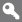X

to continue to ilmkidunya.com

X

to continue to ilmkidunya.com

X

Register Type

Please Provide following information to Register

•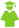Student
•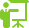Tutor
•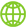Consultant
•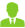Employer

CSS Examination Syllabus - Pure Mathematics

Pure Mathematics ( Total Marks - 200 )

Paper I ( Total Marks - 100 )

Note: Candidate will be asked to attempt total five questions including one compulsory (objective type) question. They will attempt at least two questions from each Section. Short note within the question (without choice) can also be given.

• Candidates will he asked to attempt three questions from Section A and two questions from section B.

Section A

Modern Algebra

• Groups, subgroups, Languages Theorem, cyclic groups, normal sub groups, quotient groups, Fundamental theorem of homomorphism, Isomorphism theorems of groups, Inner automorphisms, Conjugate elements, conjugate subgroups, Commutator subgroups.
• Rings, Subrings, Integral domains, Quotient fields, Isomorphism theorems, Field extension and finite fields.
• Vector spaces, Linear independence, Bases, Dimension of a finitely generated space, Linear transformations, Matrices and their algebra, Reduction of matrices to their echelon form, Rank and nullity of a linear transformation.
• Solution of a system of homogeneous and non-homogeneous linear equations, Properties of determinants, Cayley-Hamilton theorem, Eigenvalues and eigenvectors, Reduction to canonical forms, specially diagonalisation.

Section B

Geometry

• Conic sections in Cartesian coordinates, Plane polar coordinates and their use to represent the straight line and conic sections, (artesian and spherical polar coordinates in three dimensions, The plane, the sphere, the ellipsoid, the paraboloid and the hyperbiloid in Cartesian and spherical polar coordinates.
• Vector equations for Plane and for space-curves. The arc length. The osculating plane. The tangent, normal and binormal, Curvature and torsion, Serre-Frenet's formulae, Vector equations for surfaces, The first and second fundamental forms, Normal, principal, Gaussian and mean curvatures,

Paper II ( Total Marks - 100 )

Candidates will be asked to attempt any three questions from Section A and two questions from Section B.

Section A

Calculus and Real Analysis

• Real Numbers, Limits, Continuity, Differentiabiliry, Indefinite integration, Mean value theorems, Taylor's theorem, Indeterminate forms, Asymptotes. Curve tracing, Definite integrals, Functions of several variables, Partial derivatives. Maxima and minima Jacobians, Double and triple integration (techniques only). Applications of Beta and Gamma func tions. Areas and Volumes. Riemann-Stieltje's integral, Improper integrals and their conditions of existences, Implicit function theorem, Absolute and conditional convergence of series of real terms, Rearrangement of series, Uniform convergence of series,
• Metric spaces, Open and closed spheres, Closure, Interior and Exterior of a set. Sequences in metric space, Cauchy sequence convergence of sequences, Examples, Complete metric spaces, Continuity in metric spaces, Properties of continuous functions,

Section B

Complex Analysis

Function of a complex variable: Demoiver's theorem and its applications, Analytic functions, Cauchy's theorem, Cauchy's integral formula, Taylor's and Laurent's series, Singularities, Cauchy residue theorem and contour integration, Fourier series and Fourier transforms, Analytic continuation.

•
• Title
• Author
• 1
• Kaplan, W.
• 2
• Analytical Function Theory Vol. I
• Hille, E.
• 3
• An Introduction to Differential Geometry
• Wilmore, T.S.
• 4
• Complex Analysis
• Goodstein, G.R.G.
• 5
• Calculus with Analytical Geometry
• Yusuf, S.M.
• 6
• Differential Geometry of Three Dimensions
• Weatherburn, C.E.
• 7
• Elements of Complex Analysis
• Pennisi, L.L.
• 8
• Theory of Groups
• Theory of Groups
• 9
• Mathematical Methods
• Yusuf, S.M.
• 10
• Mathematical Analysis
• Apostal, T.M.
• 11
• Principles of Mathematical Analysis
• Rudin, W.
• 12
• The Theory of Groups
• Macdonald, I.N.
• 13
• Topics in Algebra
• Herstein, I.N.

Check other Subjects and Syllabus below:

Compulsory Subjects

General Knowledge

Optional Subjects:

(subjects carrying a total of 600 marks to be selected)

Group A

Subjects carrying not more than 200 marks can be opted.
Note: Business Administration cannot be opted in combination with Public Administration

Group B

Subject/s carrying not more than 200 marks can be opted.

Group C

Subjects carrying not more than 200 marks can be opted.

Group D (Science Subjects )

Subject/s carrying not more than 200 marks can be opted.

Group E (History Subjects )

Subjects carrying not more than 200 marks can be opted.

Group F

Subject/s carrying not more than 200 marks can be opted.
Note: International Law CANNOT BE opted in combination with International Relations.

Group G

Subjects carrying not more than 200 marks can be opted.

Group H (Regional Languages )

Subject/s carrying not more than 200 marks can be opted.
Regional Languages: Not more than one of the 4 subjects can be opted.

Group I (National and Foreign Languages )

Subjects carrying not more than 200 marks can be opted.
National/Foreign/Classical Languages: Not more than one of 4 subjects can be opted. Note: Urdu can not be combined with Regional Languages.